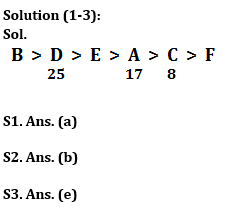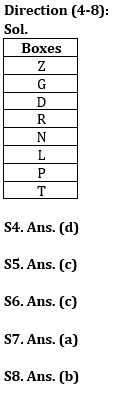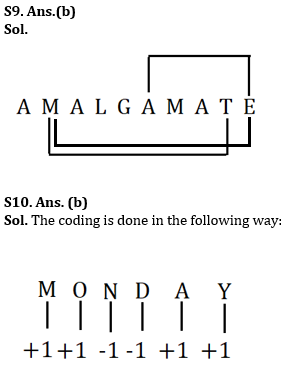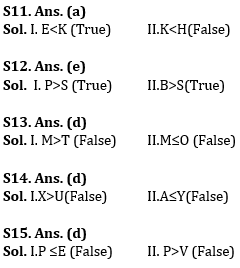Latest Banking jobs   »   Reasoning Ability Quiz For ESIC- UDC,...

# Reasoning Ability Quiz For ESIC- UDC, Steno, MTS Prelims 2022- 9th January

Directions (1-3): Study the following information carefully and answer the questions given below:

Q1. What may be the possible number of books read by E?
(a) 18
(b) 26
(c) 15
(d) 12
(e) 10

Q2. How many persons read more books than E?
(a) None
(b) Two
(c) Four
(d) Five
(e) Three

Q3. Find the sum of number of books read by A and D?
(a) 33
(b) 40
(c) 38
(d) 44
(e) 42

Directions (4-8): Study the following information carefully and answer the questions given below:
Eight boxes are kept one above the other. More than five boxes are kept above box P. Box D is kept immediately above box R and immediately below box G. Two boxes are kept between box R and box Z. Box N is kept below Z and above box L. Box T is kept below box L but not immediate below. At least four boxes are kept below box R.

Q4. Which of the following is not true?
(a)Box P is kept above box T
(b)Box T is kept at the bottommost position
(c)There are two boxes kept below box L
(d) Box R is kept immediately above box L
(e)All are incorrect

Q5. Number of boxes kept below box G is same as number of boxes kept above_______?
(a)N
(b)L
(c)P
(d)R
(e)None of these

Q6. How many boxes are kept above box Z?
(a)Two
(b)Three
(c)None
(d)Five
(e)Four

Q7. Which box is kept exactly between box N and box P?
(a)L
(b)T
(c)Z
(d)R
(e)None of these

Q8. If box P is related to box L, box N is related to box R, then in the same manner box G is related to _________?
(a)D
(b)Z
(c)R
(d)T
(e)None of these

Q9. How many pairs of letters are there in the word ‘AMALGAMATE’, each of which have as many letters between them in the word as they have between them in the English alphabet (From both forward and backward directions)?
(a) one
(b) three
(c) two
(d) four
(e) None

Q10.In a certain code “MONDAY” is coded as “NPMCBZ” and “SUNDAY” is coded as “TVMCBZ”, then what will be the code for the word “FRIDAY”?
(a)ESHCBZ
(b)GSHCBZ
(c)FSHDBZ
(d)ESICBZ
(e)EQHCBZ

Directions (11-15): In these questions, relationship between different elements is shown in the statements. The statements are followed by two conclusions.
(a) If only conclusion I is true
(b) If only conclusion II is true
(c) If either conclusion I or II is true
(d) If neither conclusion I nor II is true
(e) If both conclusions I and II are true

Q11.Statements: E ≤ F > C ≥ B; F < K≤ T; T ≤ D > H
Conclusions:
I. E<K
II.K<H

Q12.Statements: M>N<P≥ B; S< B≤ T; K >G ≤ S
Conclusions:
I. P>S
II.B>S

Q13.Statements: M<N>X= B; N≤ T=O; T >G ≤ S
Conclusions:
I. M>T
II.M≤O

Q14.Statements: W<X=Y>Z; B=C≤X; A=Y≥U
Conclusions:
I.X>U
II.A≤Y

Q15.Statements: Q ≥ S, P≤X; E ≤ X = V < S
Conclusions:
I.P ≤E
II. P>V

Solutions#### Congratulations!Union Budget 2023-24: Free PDF